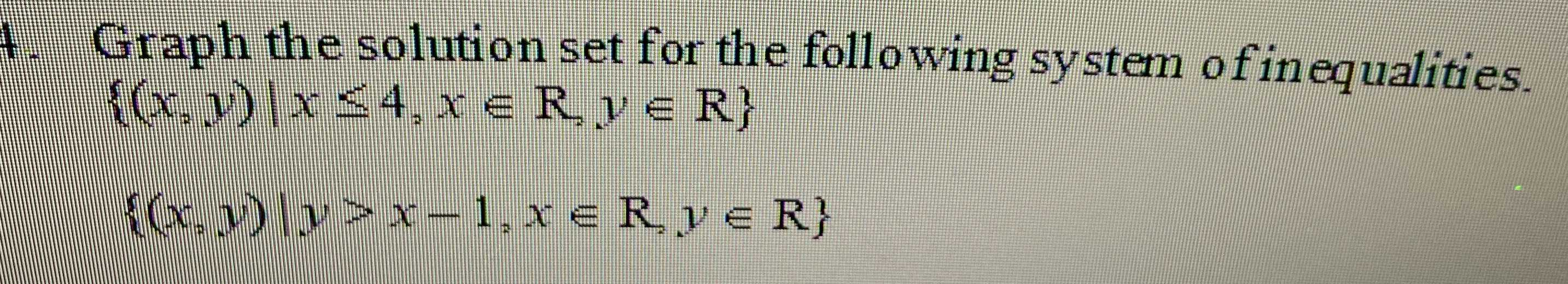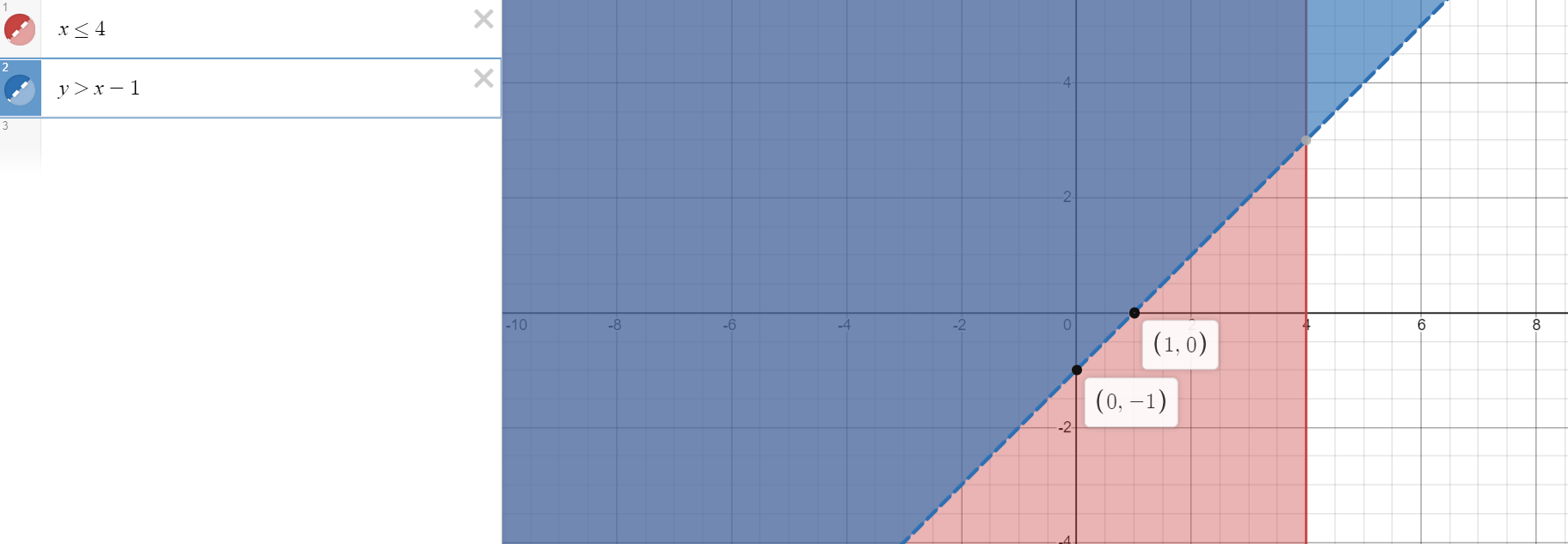### ¿Todavía tienes preguntas de matemáticas?

Pregunte a nuestros tutores expertos
Algebra
Pregunta4. Graph the solution set for the following system of inequalities.

$$\{ ( x , y ) | x \leq 4 , x \in R , y \in R \}$$ $$\{ ( x , y ) | y > x - 1 , x \in R , y \in R \}$$